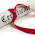## How to

When solving these problems, learning by doing is much better than learning by reading. I encourage to you read only as far in the solution as you need, then trying to solve the problem. If you get stuck, try reading a little further. And of course, let me know if you find a better solution!

## Wednesday, May 16, 2012

### Fibonacci (today) + Fibonacci (yesterday)

Problem:  Write a recursive function to prints the xth Fibonacci number which can do it in linear time

int finNum(int num) {...}

Solution:

Yesterday, we printed the the nth Fibonacci number with a very simple algorithm, but wow, was it slow.  The running time was O(2^n).  Wow.  That's really, really bad, even for relatively low values of n (like 60).

Well, we have to keep it recursive, but need to do in linear time.

Let's start by taking a look at yesterday's code:

int fibNum(int num) {

/* Check for the error case of negative number or 0 */
if (num <= 0) return ERROR;

/* Now, enter the two base cases */
if (num == 1) return 0;
else if (num == 2) return 1;

/* and now, recursive call to figure out the number */
else return (fibNum(num-1) + fibNum(num-2));
}

This code makes spawns to new calls, which each spawns two more threads as it recurs on itself.  This leads to the really horrible run time for the code.  Clearly, we have to do better

Also, this is very, very repetitive, as we continually call fibNum on the same number.  For example, in yesterday's example, we would call fibNum on fibNum of 1 or 2 as much as O(2^n).  Other numbers are called significantly too.  This seems unnecessary

Think about how you could change the number of times that fibNum is called for each number.

One solution might appear to build a data structure, that you would populate the first time you get a fibNum, then could check if you had already called fibNum on that number, and recur if you did not.  This would certainly work.  You could do this by storing an array, which would allow easy lookup by index, and then only recur if it was undefined.

Good solution, but we can do even better, without additional memory.

To provide a hint, take a look at the return statement.  The Fibonacci function requires the two previous values, but we do not keep track of them, so we have to recur each time to get them.  Think about a recursive function which kept track of the two previous values each time.

In fact, if we could do this, we would not have to go ahead and spawn two threads each time.  To do this recursively, we would need a function which kept track of the two previous values, and then would update on each recursive call, so that it would 'shift' and move the previous value back two, and our current value back one.

The base case here would be reaching the second value, or num.  And we can imagine num getting smaller until we reach the base case, while we build up the value for num.

This is a slightly more complicated function, but much more efficient.  It is a recursive solution that keeps track of the two previous values each time, and we seed a helper funciton with the first two values, 0 and 1.

Now, using this recursive insight, we can go ahead and code the problem, making sure that check bases cases.

int fibNum(int num) {

/* Check for the error case of negative number or 0 */
if (num <= 0) return ERROR;

/* Now, enter the two base cases */
if (num == 1) return 0;
else if (num == 2) return 1;

/* and now, recursive call to figure out the number */
else return (fibHelper(num, 0, 1);
}

int fibHelper(int num, int one_prev, int two_prev) {

if(n == 1) return two_prev;
return fibHelper(n - 1, two_prev, one_prev  + two_prev);
}

Now, let's try an example, say 5.  Yup.  It works!

So, what is the run time of this function?  We will make the same number of calls to fibHelper as num, so it is O(n), a WHOLE lot faster that the previous, simple implementation.

We can also drop one of the base cases now, for simplicity, as we don't need to treat num=2 as a special case anymore, which give us (fibHelper remains the same):

int fibNum(int num) {

/* Check for the error case of negative number or 0 */
if (num <= 0) return ERROR;

/* Now, enter the two base cases */
if (num == 1) return 0;

/* and now, recursive call to figure out the number */
else return (fibHelper(num, 0, 1);
}

This is a good example of where by simply going a little further, we are able to take a disastrously inefficient function, and make it better, and why you shouldn't stop at just a 'good' solution.

/* Please let me know if you find any bugs here.  */

#### 1 comment:

1.Ace4sure is the website that deals in preparation material for the exam for many years. According to my exposure and research, this is the right platform where you can get exact MS-500 Exam Dumps.